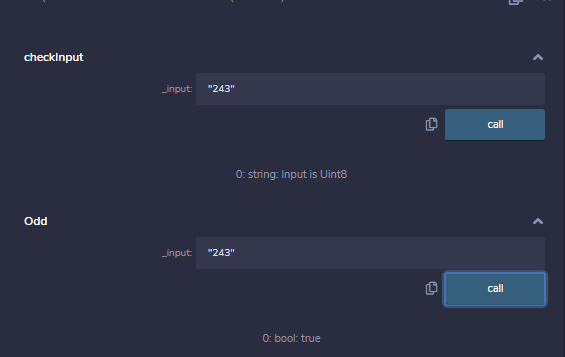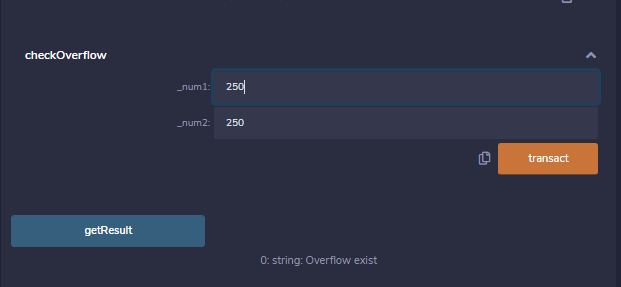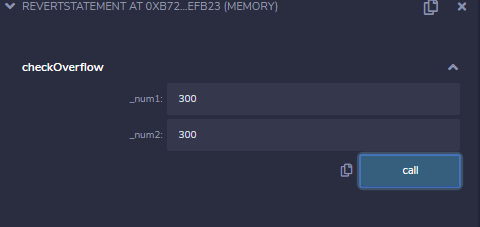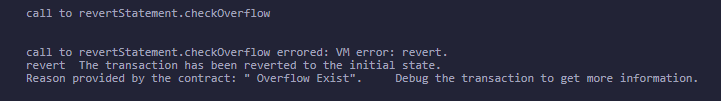Related Articles

# Solidity – Error Handling

• Last Updated : 13 Jul, 2020

Solidity has many functions for error handling. Errors can occur at compile time or runtime. Solidity is compiled to byte code and there a syntax error check happens at compile-time, while runtime errors are difficult to catch and occurs mainly while executing the contracts. Some of the runtime errors are out-of-gas error, data type overflow error, divide by zero error, array-out-of-index error, etc. Until version 4.10 a single throw statement was there in solidity to handle errors, so to handle errors multiple if…else statements, one has to implement for checking the values and throw errors which consume more gas. After version 4.10 new error handling construct assert, require, revert statements were introduced and the throw was made absolute.

### Require Statements

The ‘require’ statements declare prerequisites for running the function i.e. it declares the constraints which should be satisfied before executing the code. It accepts a single argument and returns a boolean value after evaluation, it also has a custom string message option. If false then exception is raised and execution is terminated. The unused gas is returned back to the caller and the state is reversed to its original state. Following are some cases when require type of exception is triggered :

• When require() is called with the arguments which result as false.
• When a function called by a message does not end properly.
• When a contract is created using the new keyword and the process does not end properly.
• When a codeless contract is targeted to an external function.
• When ethers are sent to the contract using the public getter method.
• When .transfer() method fails.
• When an assert is called with a condition that results in false.
• When a zero-initialized variable of a function is called.
• When a large or a negative value is converted to an enum.
• When a value is divided or modulo by zero.
• When accessing an array in an index which is too big or negative.

Example: In the below example, the contract requireStatement demonstrates how to use the ‘require statement’.

## Solidity

 `// Solidity program to ``// demonstrate require``// statement``pragma solidity ^0.5.0;`` ` `// Creating a contract``contract` `requireStatement {``    ` `    ``// Defining function to ``    ``// check input``    ``function checkInput(``      ``uint _input) ``public` `view returns(``      ``string memory){``        ``require(_input >= 0, ``"invalid uint8"``);``        ``require(_input <= 255, ``"invalid uint8"``);``         ` `        ``return` `"Input is Uint8"``;``    ``}``    ` `    ``// Defining function to ``    ``// use require statement``    ``function Odd(uint _input) ``public` `view returns(``bool``){``        ``require(_input % 2 != 0);``        ``return` `true``;``    ``}``}`

Output :### Assert Statement

Its syntax is similar to the require statement. It returns a boolean value after the evaluation of the condition. Based on the return value either the program will continue its execution or it will throw an exception. Instead of returning the unused gas, the assert statement consumes the entire gas supply and the state is then reversed to the original state. Assert is used to check the current state and function conditions before the execution of the contract. Below are some cases with assert type exceptions :

• When an assert is called with a condition that results in false.
• When a zero-initialized variable of a function is called.
• When a large or a negative value is converted to an enum.
• When a value is divided or modulo by zero.
• When accessing an array in an index which is too big or negative.

Example: In the below example, the contract assertStatement demonstrates how to use an ‘assert statement’.

## Solidity

 `// Solidity program to``// demonstrate assert ``// statement``pragma solidity ^0.5.0;`` ` `// Creating a contract``contract` `assertStatement {``   ` `    ``// Defining a state variable  ``    ``bool` `result;`` ` `    ``// Defining a function ``    ``// to check condition``    ``function checkOverflow(``      ``uint _num1, uint _num2) ``public` `{``        ``uint8 sum = _num1 + _num2;``        ``assert``(sum<=255);``        ``result = ``true``;``    ``}``    ` `    ``// Defining a function to ``    ``// print result of assert``    ``// statement``    ``function getResult() ``public` `view returns(string memory){``         ``if``(result == ``true``){``             ``return` `"No Overflow"``;``         ``}``         ``else``{``             ``return` `"Overflow exist"``;``         ``}``    ``}``}`

Output :### Revert Statement

This statement is similar to the require statement. It does not evaluate any condition and does not depends on any state or statement. It is used to generate exceptions, display errors, and revert the function call. This statement contains a string message which indicates the issue related to the information of the exception. Calling a revert statement implies an exception is thrown, the unused gas is returned and the state reverts to its original state.  Revert is used to handle the same exception types as require handles, but with little bit more complex logic.

Example: In the below example, the contract revertStatement demonstrates the ‘revert statement’.

## Solidity

 `// Solidity program to ``// demonstrate revert``// statement``pragma solidity ^0.5.0;`` ` `// Creating a contract``contract` `revertStatement {``   ` `    ``// Defining a function``    ``// to check condition``    ``function checkOverflow(``      ``uint _num1, uint _num2) ``public` `view returns(``      ``string memory, uint) {``        ``uint sum = _num1 + _num2;``         ``if``(sum < 0 || sum > 255){``             ``revert(``" Overflow Exist"``);``         ``}``         ``else``{``             ``return` `(``"No Overflow"``, sum);``         ``}``          ` `    ``}``     ` `}``     ` `}`

Output :My Personal Notes arrow_drop_up